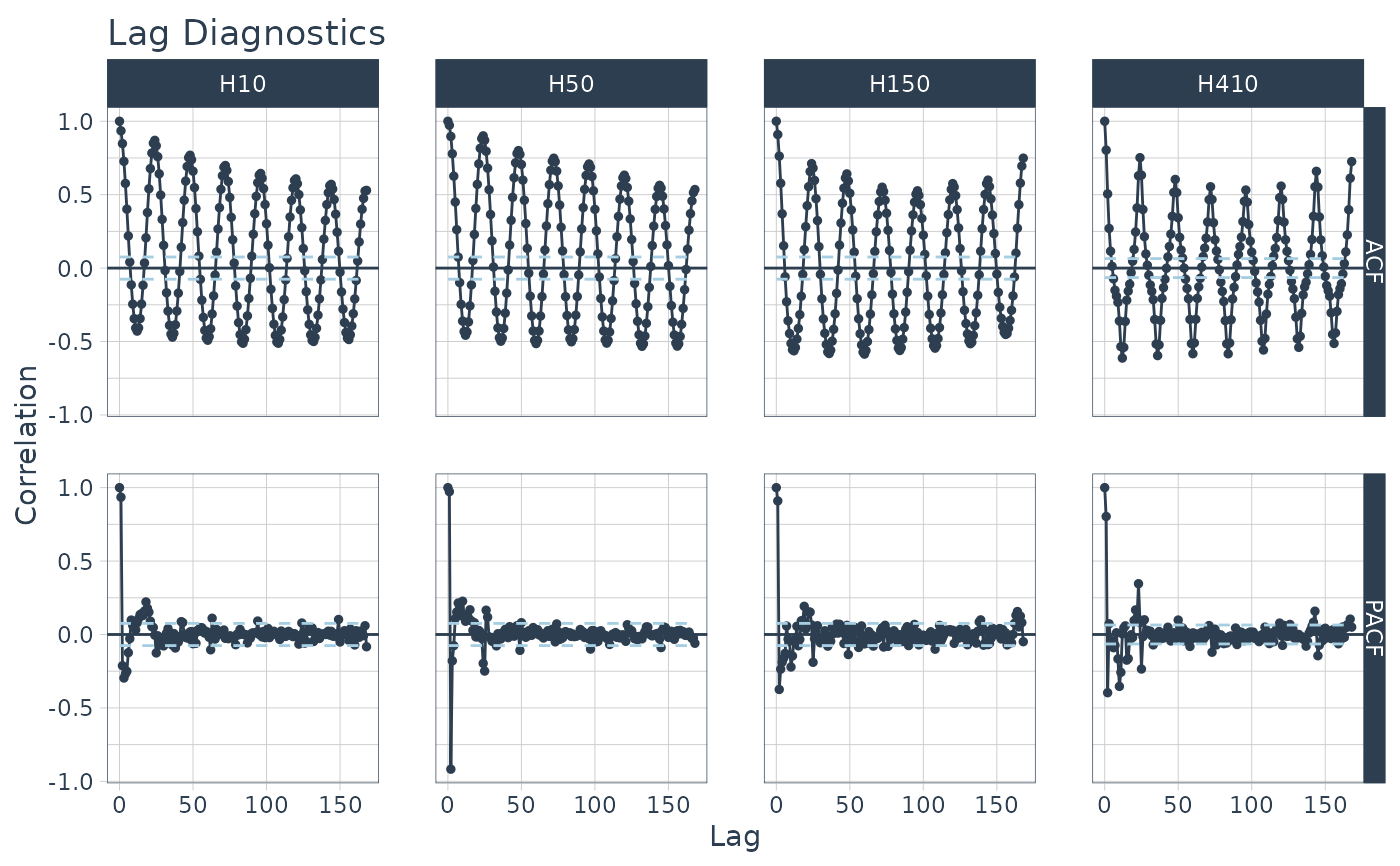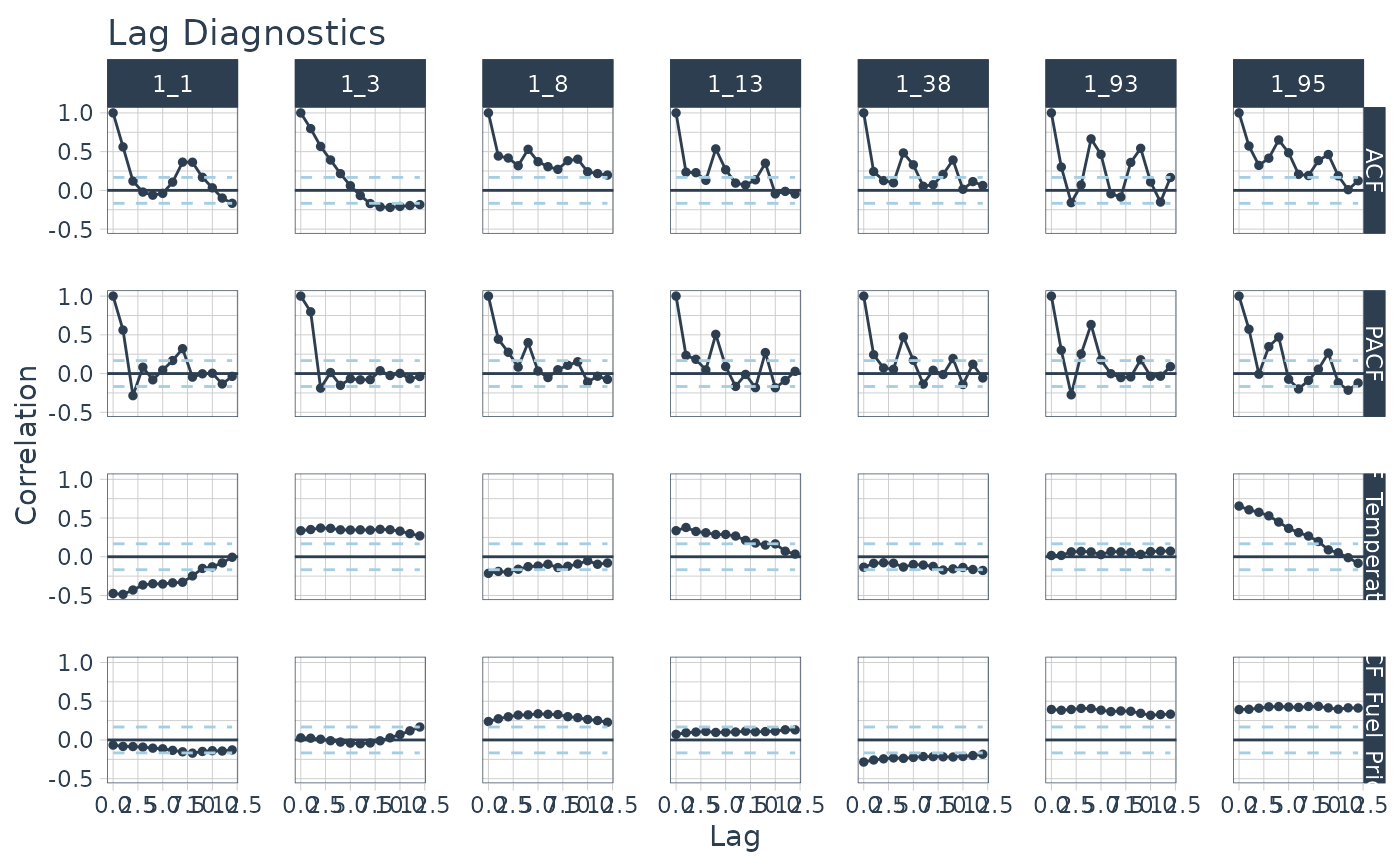Returns the ACF and PACF of a target and optionally CCF's of one or more lagged predictors in interactive plotly plots. Scales to multiple time series with group_by().

## Usage

plot_acf_diagnostics(
.data,
.date_var,
.value,
.ccf_vars = NULL,
.lags = 1000,
.show_ccf_vars_only = FALSE,
.show_white_noise_bars = TRUE,
.facet_ncol = 1,
.facet_scales = "fixed",
.line_color = "#2c3e50",
.line_size = 0.5,
.line_alpha = 1,
.point_color = "#2c3e50",
.point_size = 1,
.point_alpha = 1,
.x_intercept = NULL,
.x_intercept_color = "#E31A1C",
.hline_color = "#2c3e50",
.white_noise_line_type = 2,
.white_noise_line_color = "#A6CEE3",
.title = "Lag Diagnostics",
.x_lab = "Lag",
.y_lab = "Correlation",
.interactive = TRUE,
.plotly_slider = FALSE
)

## Arguments

.data

A data frame or tibble with numeric features (values) in descending chronological order

.date_var

A column containing either date or date-time values

.value

A numeric column with a value to have ACF and PACF calculations performed.

.ccf_vars

Additional features to perform Lag Cross Correlations (CCFs) versus the .value. Useful for evaluating external lagged regressors.

.lags

A sequence of one or more lags to evaluate.

.show_ccf_vars_only

Hides the ACF and PACF plots so you can focus on only CCFs.

.show_white_noise_bars

Shows the white noise significance bounds.

.facet_ncol

Facets: Number of facet columns. Has no effect if using grouped_df.

.facet_scales

Facets: Options include "fixed", "free", "free_y", "free_x"

.line_color

Line color. Use keyword: "scale_color" to change the color by the facet.

.line_size

Line size

.line_alpha

Line opacity. Adjust the transparency of the line. Range: (0, 1)

.point_color

Point color. Use keyword: "scale_color" to change the color by the facet.

.point_size

Point size

.point_alpha

Opacity. Adjust the transparency of the points. Range: (0, 1)

.x_intercept

Numeric lag. Adds a vertical line.

.x_intercept_color

Color for the x-intercept line.

.hline_color

Color for the y-intercept = 0 line.

.white_noise_line_type

Line type for white noise bars. Set to 2 for "dashed" by default.

.white_noise_line_color

Line color for white noise bars. Set to tidyquant::palette_light() "steel blue" by default.

.title

Title for the plot

.x_lab

X-axis label for the plot

.y_lab

Y-axis label for the plot

.interactive

Returns either a static (ggplot2) visualization or an interactive (plotly) visualization

.plotly_slider

If TRUE, returns a plotly x-axis range slider.

## Value

A static ggplot2 plot or an interactive plotly plot

## Details

Simplified ACF, PACF, & CCF

We are often interested in all 3 of these functions. Why not get all 3+ at once? Now you can.

• ACF - Autocorrelation between a target variable and lagged versions of itself

• PACF - Partial Autocorrelation removes the dependence of lags on other lags highlighting key seasonalities.

• CCF - Shows how lagged predictors can be used for prediction of a target variable.

Lag Specification

Lags (.lags) can either be specified as:

• A time-based phrase indicating a duraction (e.g. 2 months)

• A maximum lag (e.g. .lags = 28)

• A sequence of lags (e.g. .lags = 7:28)

Scales to Multiple Time Series with Groups

The plot_acf_diagnostics() works with grouped_df's, meaning you can group your time series by one or more categorical columns with dplyr::group_by() and then apply plot_acf_diagnostics() to return group-wise lag diagnostics.

Special Note on Groups

Unlike other plotting utilities, the .facet_vars arguments is NOT included. Use dplyr::group_by() for processing multiple time series groups.

Calculating the White Noise Significance Bars

The formula for the significance bars is +2/sqrt(T) and -2/sqrt(T) where T is the length of the time series. For a white noise time series, 95% of the data points should fall within this range. Those that don't may be significant autocorrelations.

• Visualizing ACF, PACF, & CCF: plot_acf_diagnostics()

• Visualizing Seasonality: plot_seasonal_diagnostics()

• Visualizing Time Series: plot_time_series()

## Examples

library(dplyr)
library(ggplot2)
library(timetk)

# Apply Transformations
# - Differencing transformation to identify ARIMA & SARIMA Orders
m4_hourly %>%
group_by(id) %>%
plot_acf_diagnostics(
date, value,               # ACF & PACF
.lags = "7 days",          # 7-Days of hourly lags
.interactive = FALSE
)# Apply Transformations
# - Differencing transformation to identify ARIMA & SARIMA Orders
m4_hourly %>%
group_by(id) %>%
plot_acf_diagnostics(
date,
diff_vec(value, lag = 1), # Difference the value column
.lags        = 0:(24*7),   # 7-Days of hourly lags
.interactive = FALSE
) +
ggtitle("ACF Diagnostics",  subtitle = "1st Difference")
#> diff_vec(): Initial values: 513
#> diff_vec(): Initial values: 39325
#> diff_vec(): Initial values: 45
#> diff_vec(): Initial values: 153# CCFs Too!
walmart_sales_weekly %>%
select(id, Date, Weekly_Sales, Temperature, Fuel_Price) %>%
group_by(id) %>%
plot_acf_diagnostics(
Date, Weekly_Sales,                        # ACF & PACF
.ccf_vars    = c(Temperature, Fuel_Price), # CCFs
.lags        = "3 months", # 3 months of weekly lags
.interactive = FALSE
)